# Use the DATEDIF function to calculate the intervals of dates

Uploaded time: August 30, 2021 Difficulty: Intermediate# Use the DATEDIF function to calculate the intervals of dates

The DATEDIF function is mainly used to calculate days, months, or years between two dates.

Now we are going to use this table to show you the usage of this function. This table records the entry date of the companys employees. Now we want to calculate the employees year of service. We can first click cell C2 and then click Insert Function. Insert DATEDIF in the Search for Function of the dialog box. Click the OK button, and then the Function Arguments dialog box will pop up.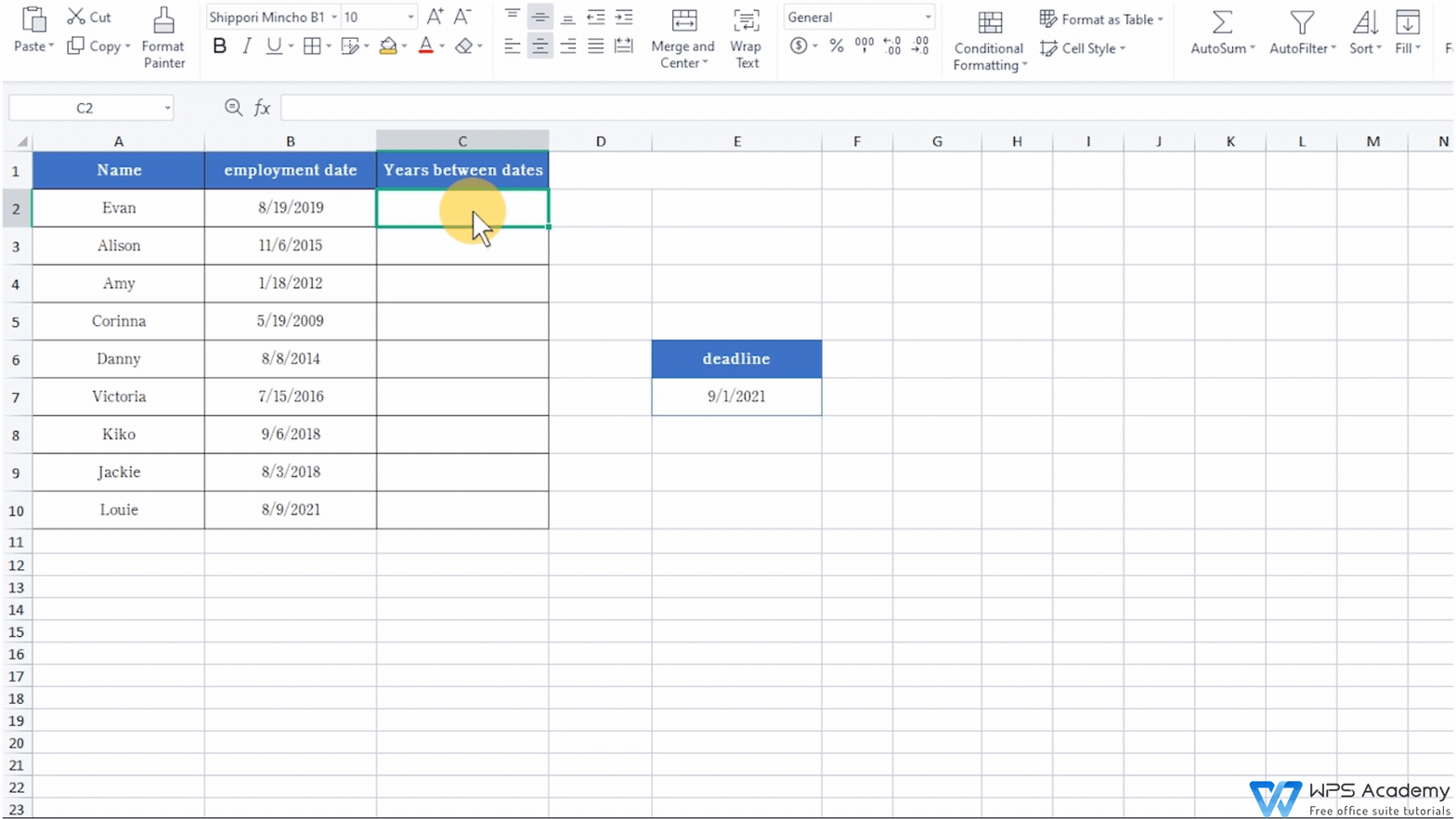Start date refers to the date we want to start with, which corresponds to the employment date data in column B of the table.  Click on cell B2 here.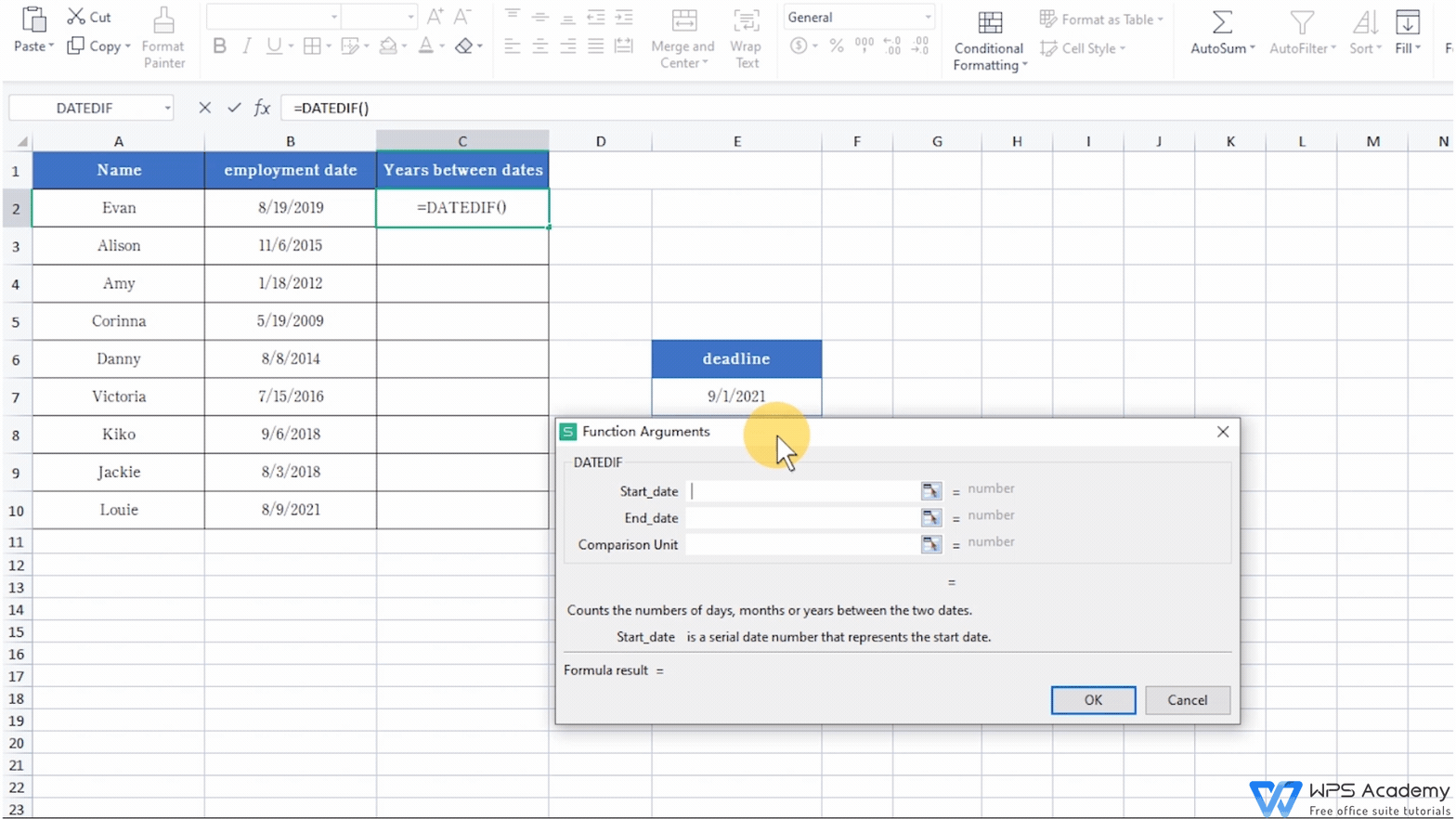End date refers to the date we want to stop the calculation, which is actually the deadline 9/1/2021 on the right side of the table. Click cell E7 here, then press F4 to add an absolute reference and lock the End date calculation location.

Please notice that the end date here should be greater than the start date.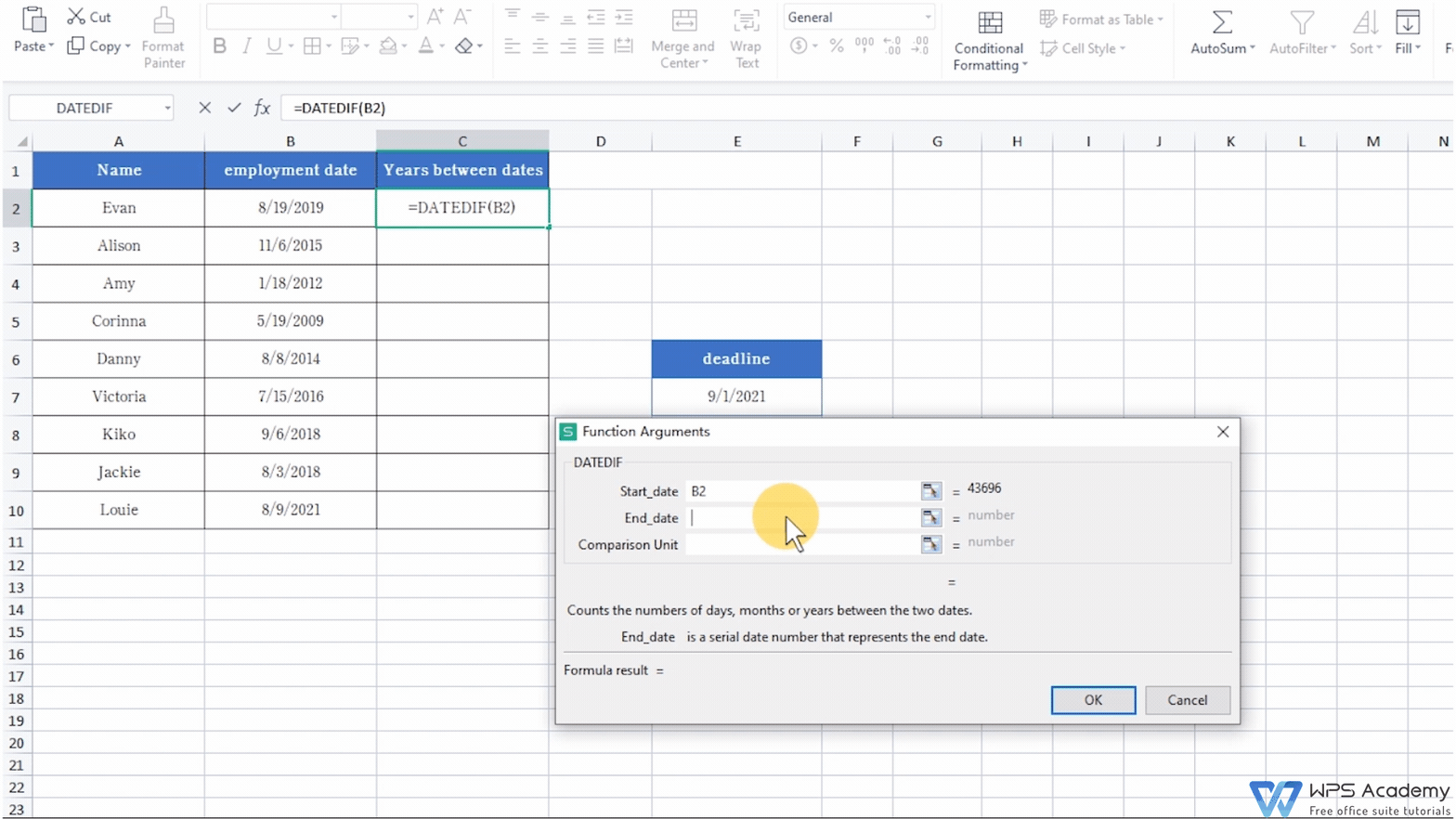Comparison Unit refers to the return type of the required information. That is to say, different parameters represent different return results. Y is the number of years between these two dates, M is the number of months between these two dates, and D is the number of days between these two dates. Remember to add double quotes when entering parameters. Enter Y here.

Now click the OK button to get the result. Then, move the mouse cursor to the lower right corner of the cell. Pull it down to get the corresponding calculation data when the cursor turns into a black cross.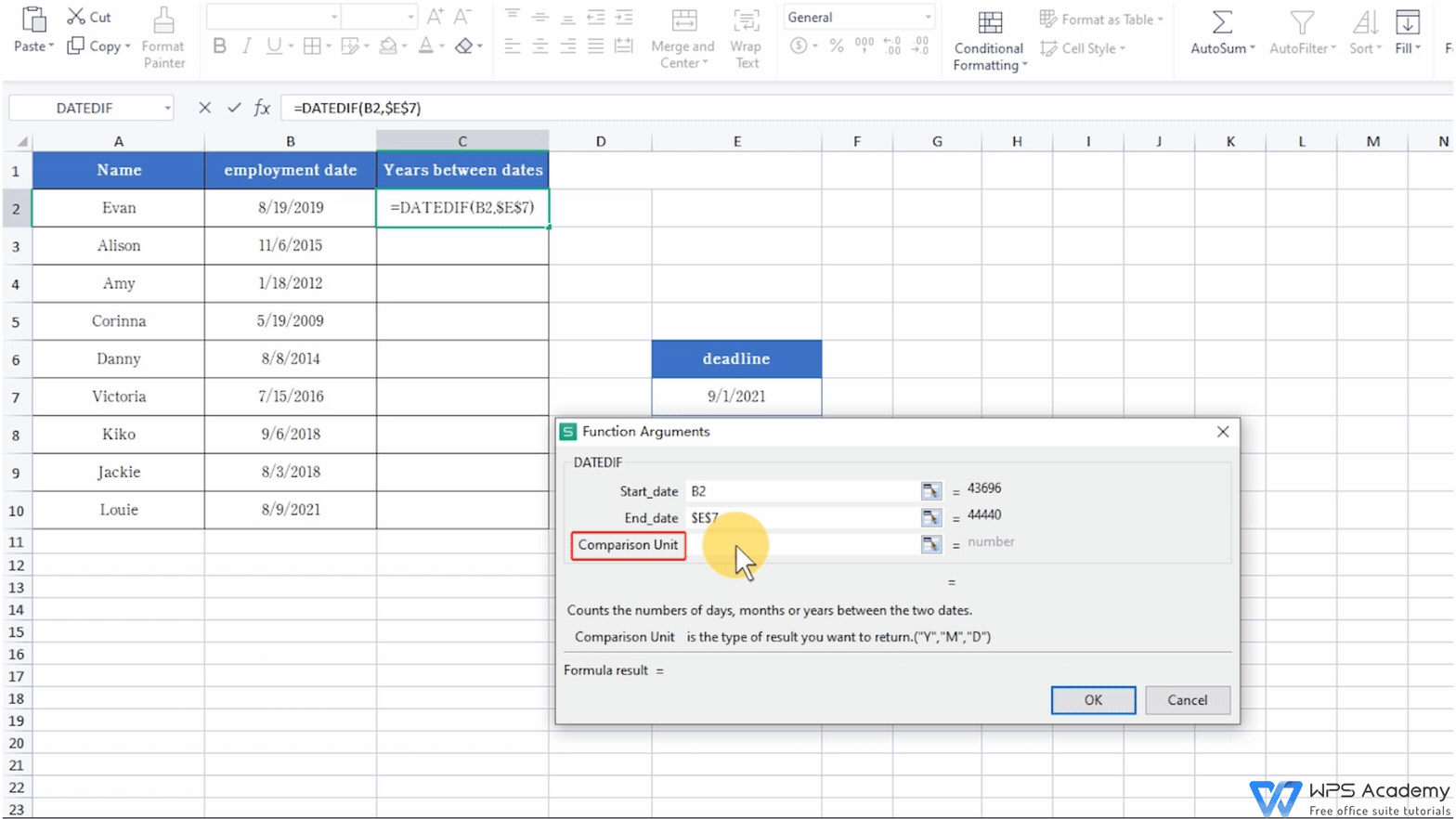At this time, we can see that the resulting data are all integers, and those digits after the decimal point are all deleted. For example, the entry time of Louie is only nearly one month. Since it's less than one year, only the number 0 is displayed.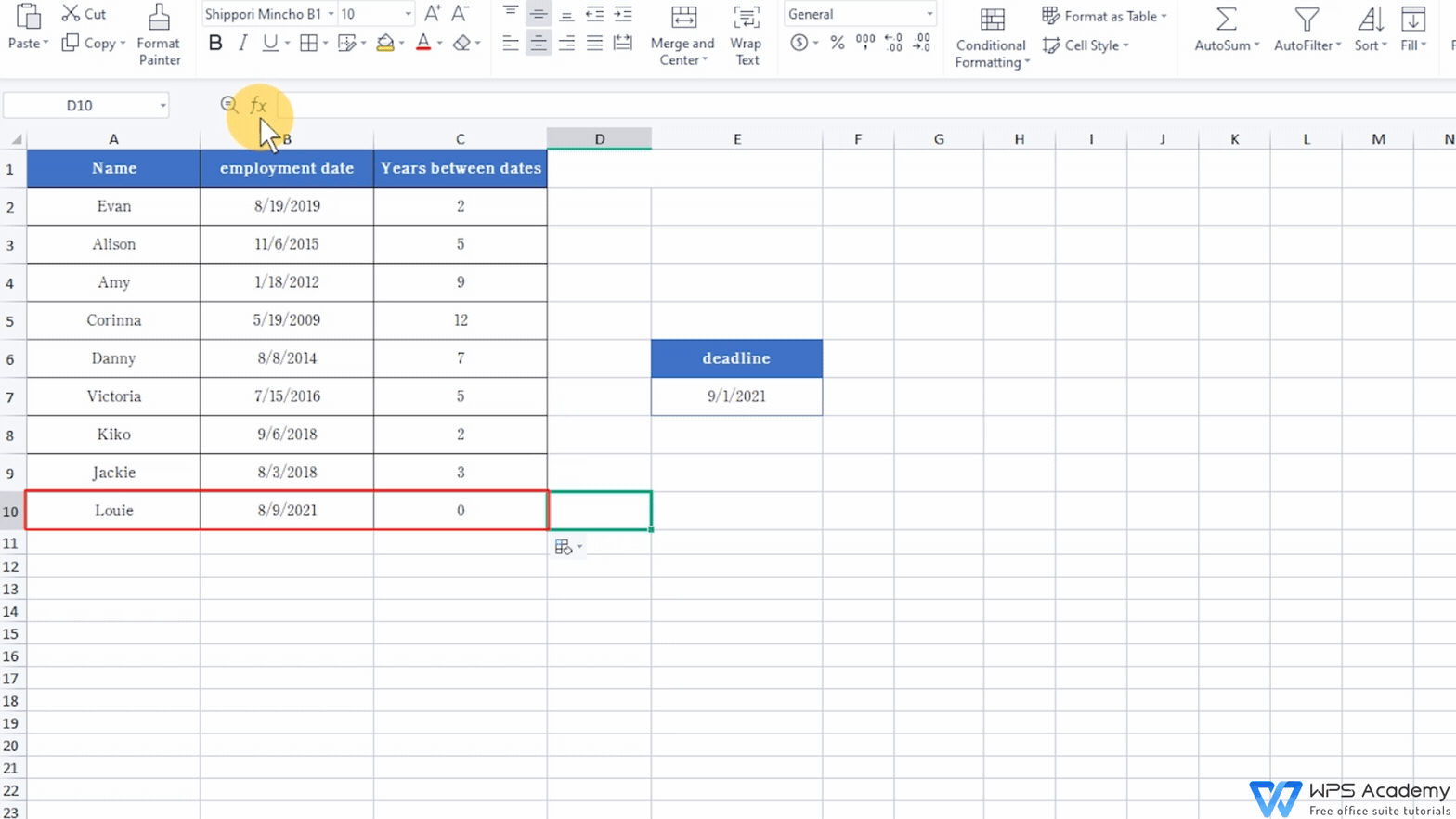In addition, we can also ignore the difference in years between the two dates and only calculate the number of days between the dates.  Let's take Louie's entry date as an example again. Click cell D10, and then click Insert Function to select the DATEDIF function. Click cell B10 for Start date, click E7 for End date, and enter YD for Comparison Unit. Now click the OK button, then we can get the number of days between August 9th and September 1st. The result is 23 days.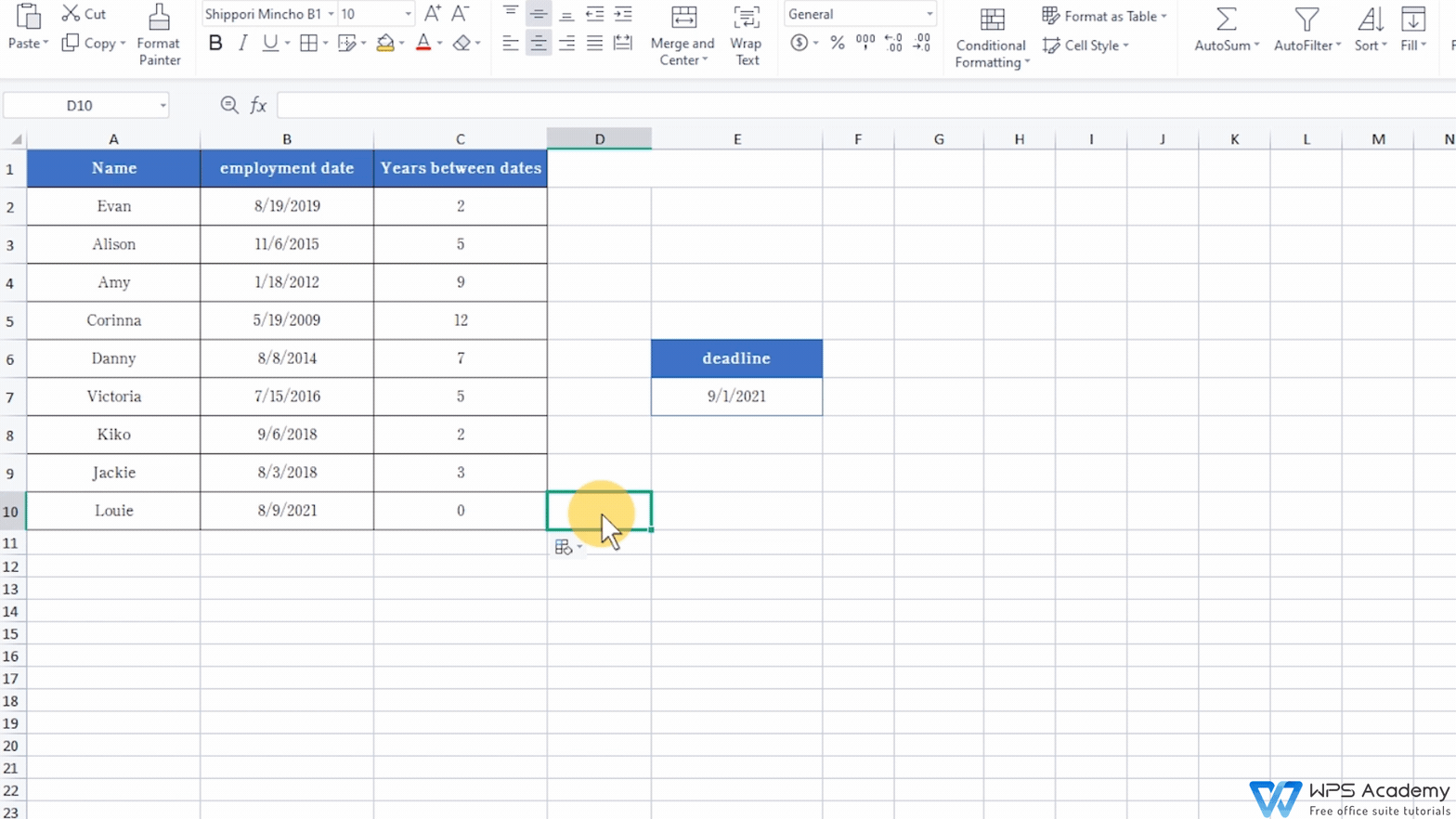Also, the parameter MD in the Comparison Unit represents the number of days between two dates while ignoring the difference in years and months between the two dates. YM means calculating the number of months between two dates while ignoring the difference in years between the two dates.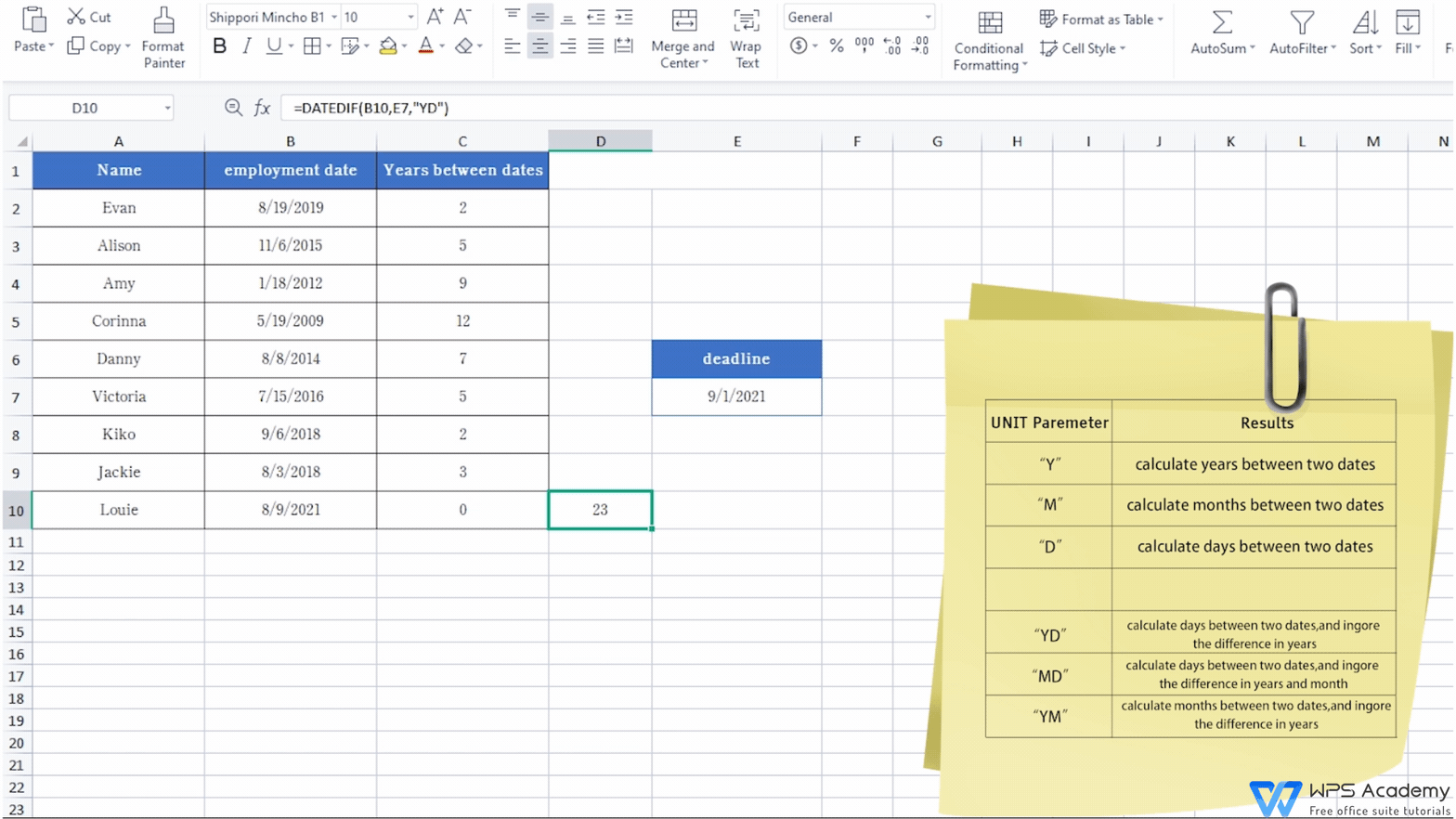Easy your work with WPS Spreadsheet like Microsoft Excel. Did you get it?